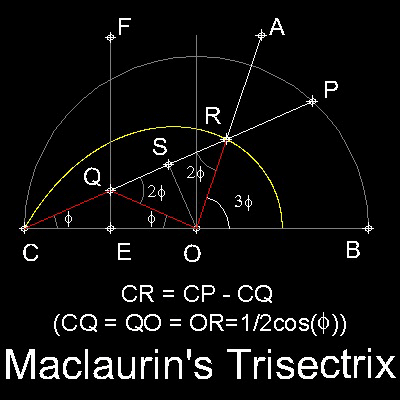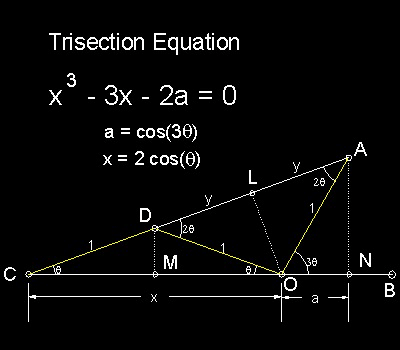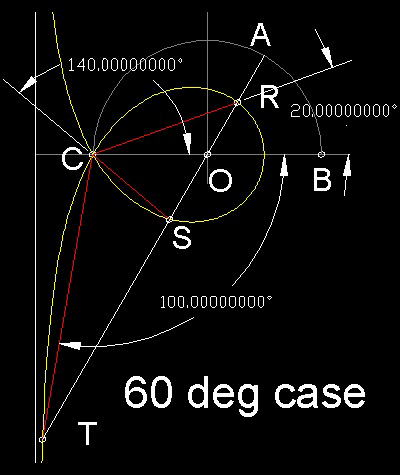Special Curves
Go to   Fun_Math Content Table   Trisecting an Angle   Special Curves

### Trisection using Special Curves

#### Trisectrix by Maclaurin

Colin Maclaurin (1698-1746) used a curve called "Trisectrix" to accomplish Angle Trisection.

His idea is shown in the figure shown below.##### Equation of Trisectrix
In polar coordinate: r = CP - CQ = 2cos(q) - 1/2cos(q)

=(2cos2q)/(2cosq) + 1/(2cosq) = 2QS + CQ = CR.

Therefore QS = SR, and OQ = OR.

******** Maclaurin_tri_desc.dwg ********
In rectangular coordinate:

Assuming temporalily that point C is the origin of X-Y axis

by replacing r by sqrt(x2 + y2) and cosq by y/sqrt(x2 + y2)

y2 = x2(3 - 2x) /(1 + 2x)

Now shifting the X-coordinate orign from C to O (distance of 1) (replacing x by x+1)

Trisectrix equation is :y2 = (x + 1)2(2x - 1) /(3 + 2x)

##### Characteristics of of Trisectrix
This curve intersects Y -axis at x = -1 and 1/2, and as angle QCO approaches 90 degree, point R approaches line x = -3/2,both up and down.

Why this curve can be used for trisecting angle becomes clear when this figure is compared with the following drawing used in deriving Trisection equation.******** trisection_equation_desc.dwg ********

#### Example of Trisection-60 degree caseAngle AOB is to be trisected.

There are 3 trisection solutions.

They are one third of

given angle q (= 60) --->RCO = 20 deg

360 + q --->180 - OCS = 140 deg

2x360 + q --->RCT = 260 deg (shown as -100 deg).

You can see the process in animation.

******** Maclaurin_60_deg.dwg ********

To create this drawing and animation:
Then from command line, type DRAW_MACLAURIN_ONLY for drawing Trisectrix curve

#### Trisection:

After drawing Trisectrix,define an anlge AOB to be trisected.

#### References

1. Dudley,Underwood :"The Trisectors" The Mathematical Association of America,1994.p 12-13

Go to   Fun_Math Content Table   Trisecting an Angle   Special Curves

All questions/suggestions should be sent to Takaya Iwamoto

Last Updated Nov 22, 2006